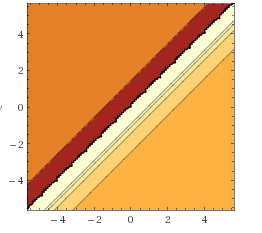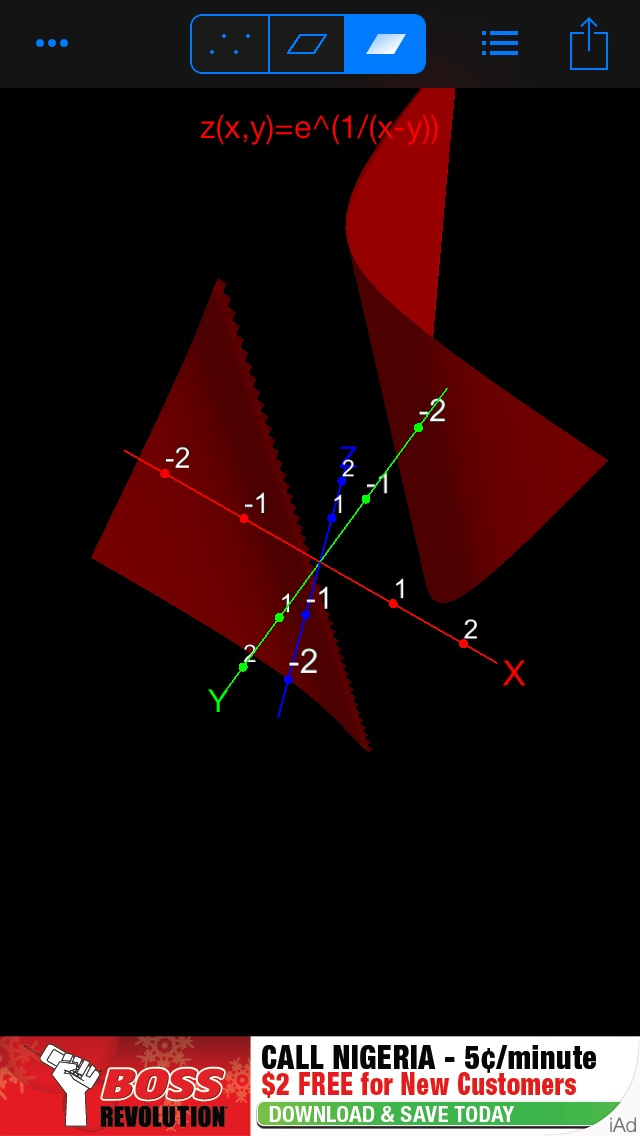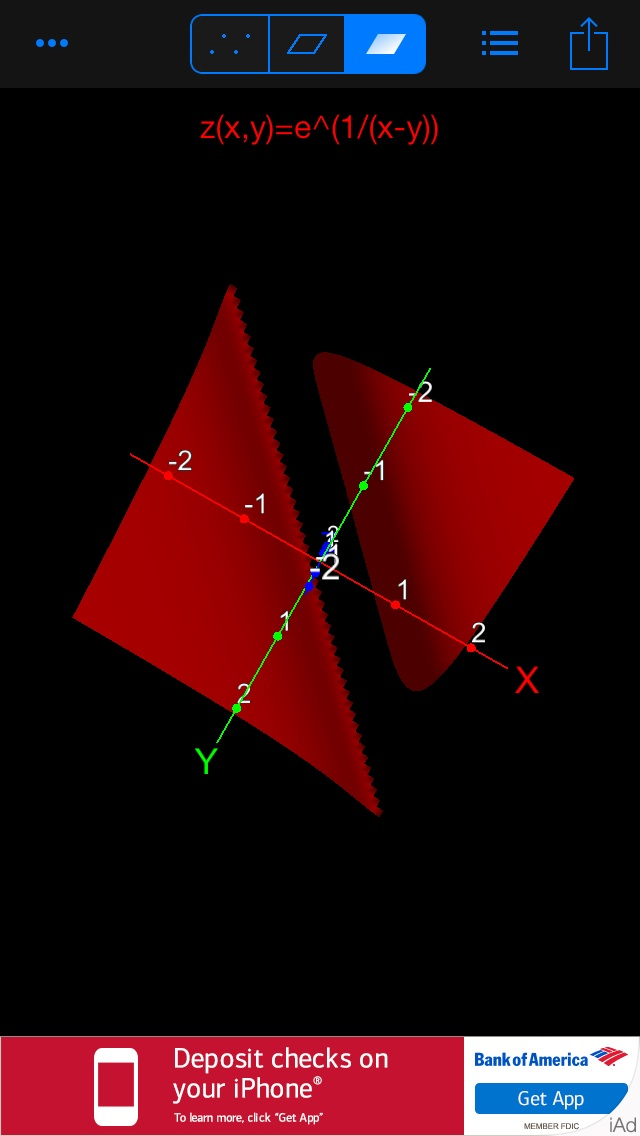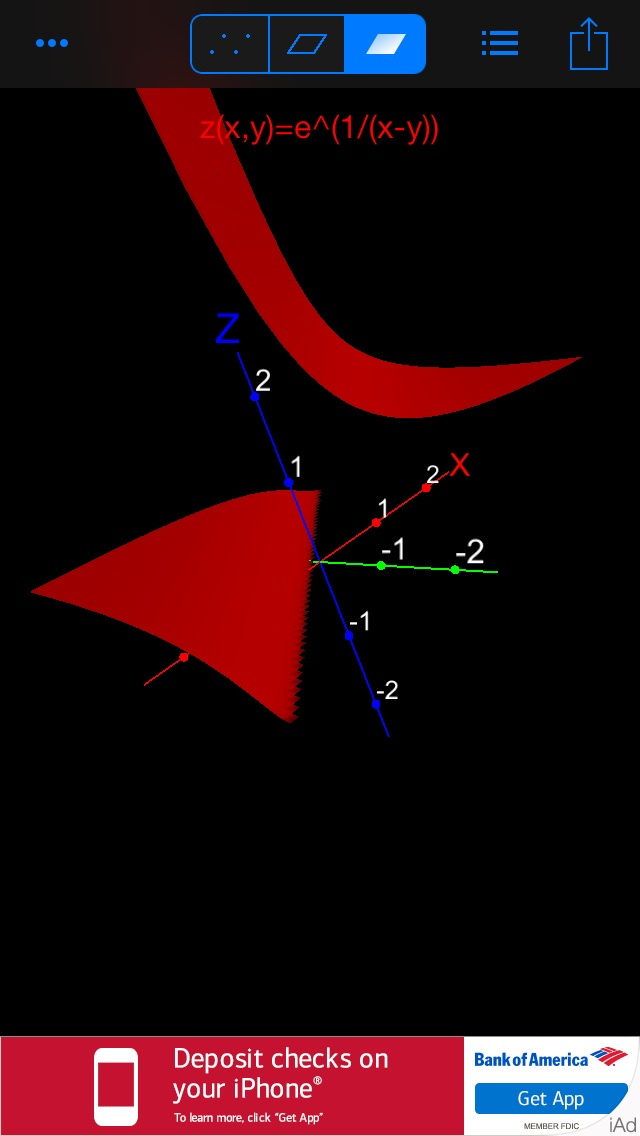# Graph the function and observe where it is discontinuous. f(x. y) = e1/(x − y)

Graph the function and observe where it is
discontinuous. f(x,y)= e^(1/(x-y)) f is discontinuous at x =????
f x ????

Domain is :

so,
f
is discontinuous at
x
= y

You should notice the
sharp edges which is the line y = x which is where the function is
discontinuous.